# Electronics and Communication Engineering - Exam Questions Papers

21.

Two discrete time systems with impulse responses h1[n] = δ[n - 1] and h2[n] = δ[n - 2] are connected in cascade. The overall impulse response of the cascaded system is

 A. δ[n - 1] + δ[n - 2] B. δ[n - 4] C. δ[n - 3] D. δ[n - 1] + δ[n - 2]

Explanation:

h1(n) → delay by 1, h2(n) → delay by 2,

h1(n) * h2(n) → delay by 3.

22.

The magnitude plot of a composite signal x(t) = e2jt + e3jt is

 A. Half wave rectified sinusoid B. Full wave rectified sinusoid C. Exponentially increasing sinusoid D. Exponentially decreasing sinusoid

Explanation:

x(t) = ej2 . 5t (e-j0.5t + e-j0 . 5t)

x(t) = 2ej2.5t cos (0.5)t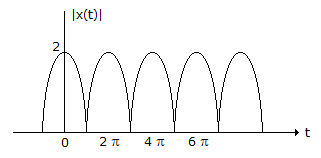The magnitude of x(t) is

|x(t)| = 2|cos (0.5)t|.

23.

A message signal given by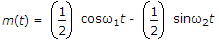is amplitude modulated with a carrier of frequency ωc to generate s(t) = [1 + m(t)] cos ωct

 A. 8.33% B. 11.11% C. 20% D. 25%

Explanation: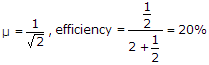.

24.

A 16 bit counter type ADC uses 1 MHz clock then its maximum conversion time is __________ .

 A. 16 μs B. 15 ms C. 6.6 ms D. 66 ms

Explanation:

Maximum conversion time of counter type ADC = (216 - 1) x 1 μs = 65.5 ms » 66 ms.

25.

A first order system will never be able to give a __________ response.

1. Band pass
2. Band reject
3. All pass
Choose the correct option

 A. 1, 2 and 3 are true B. 1 and 3 are true, 2 is false C. 1 and 2 are false, 3 is true D. 1 and 2 are true, 3 is false

Explanation:

The ideal response of a Band pass filter BRF and APF is as shown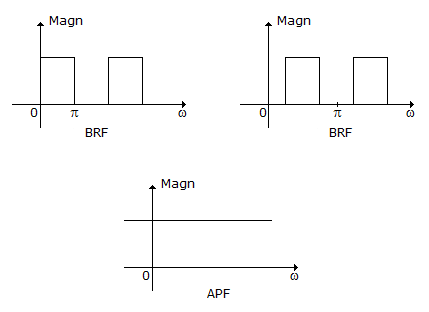So for BPF we require 0 at ω = 0, p i.e. at least 2 zeroes are required in the Transform.

Hence order has to be atleast 2.

Similarly for band reject filter order should be at least 2. Whereas APF has a constant amplitude.

Hence Zeroes in the function need not matter.

APF function is given as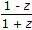Hence first order can provide APF.

#### Current Affairs 2022

Interview Questions and Answers